X=Y*（Z+10）

int age=20;

int a,b,c; //同时声明三个变量

a=b=c=1; //自右向左赋值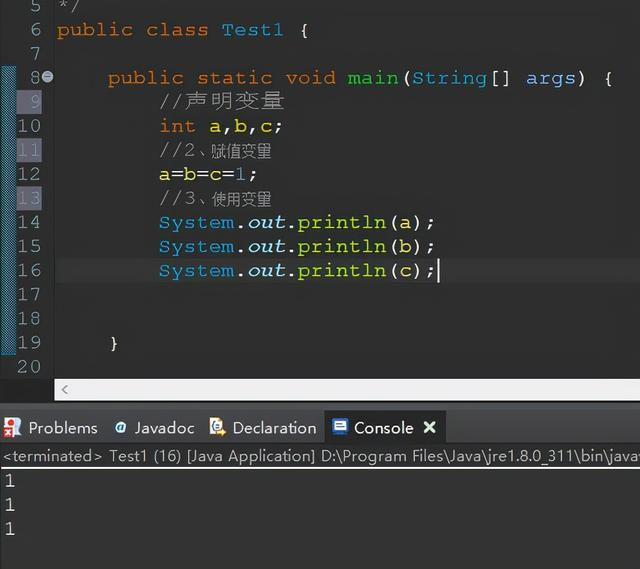Java中提供了算术运算符来实现数学上的算术运算功能

 运算符 说明 举例 + 加法运算符，求操作数的和 5+2等于7 - 减法运算符，求操作数的差 5-2等于3 * 乘法运算符，求操作数的积 5*2等于10 / 除法运算符，求操作数的商 5/2等于2，5.0/2等于2.5 % 取余（模）运算符，求操作数相除的余数 5%2等于1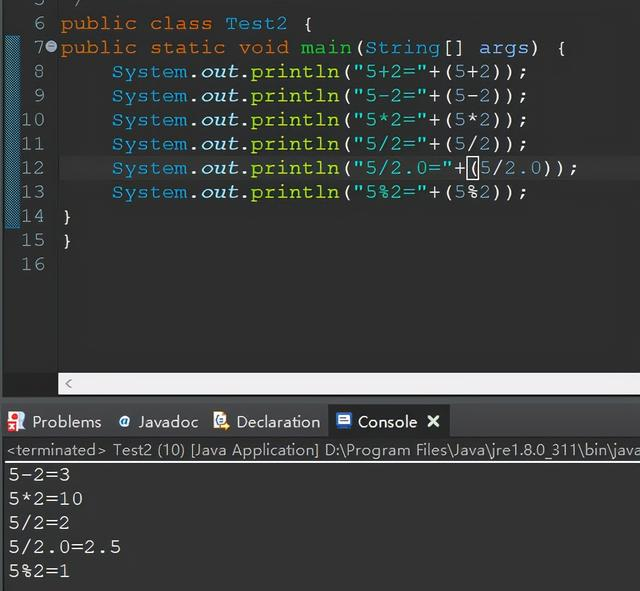运算符 说明 举例 += 加法运算 int a=8; a+2 等同于a=a+2; a=10 -= 减法运算 int a=8; a-=2 等同于a=a-2; a=6 *= 乘法运算 int a=8; a*=2 等同于a=a*2; a=16 /= 除法运算 int a=8; a/=2 等同于a=a/2; a=4 %= 模运算 int a=8; a%=2 等同于a=a%2; a=0

int a=6;

a+=a+=6;等效于a=a+(a+6);

java表达式中使用圆括号与代数中的圆括号作用相同，能增强运算符的优先级，使用圆括号环能增强程序的可读性并使计算顺序清晰。

++变量名或变量名--；

--变量名 或者变量名--；

int a=5;

int b=++a; //等效于a=a+1; int b=a;

 运算符 说明 举例 > 大于 88>100，结果为false > 小于 88<100, 结果为true >= 大于等于 50>=60, 结果为false <= 小于等于 50<=50, 结果为true == 等于 50==60，结果为false != 不等于 50！=60，结果为true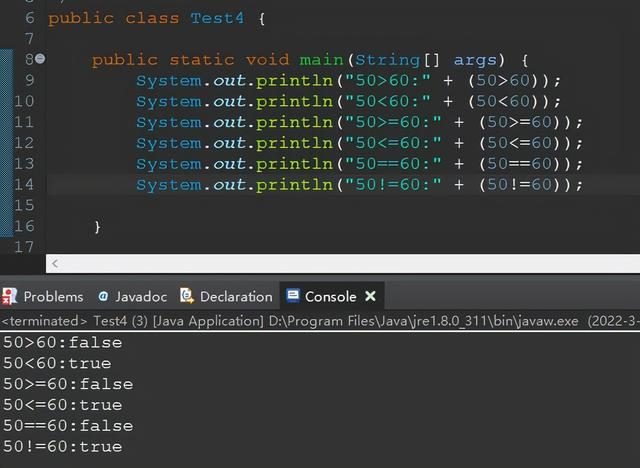运算符 说明 && 与运算，表示连接条件要同时成立 || 或运算，表示连接的条件有一个成立即可 ！ 非运算，取反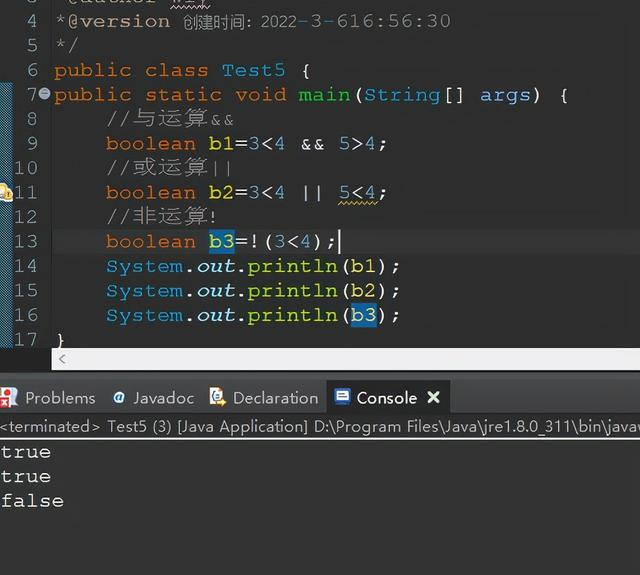“？”是java中唯一的一个三目运算符，条件表达式的结果由布尔表达式决定，如果布尔表达式的值为true，则返回表达式1的值，否则返回表达式2的值。

int score=75;

String result=score>60?"及格"："不及格"；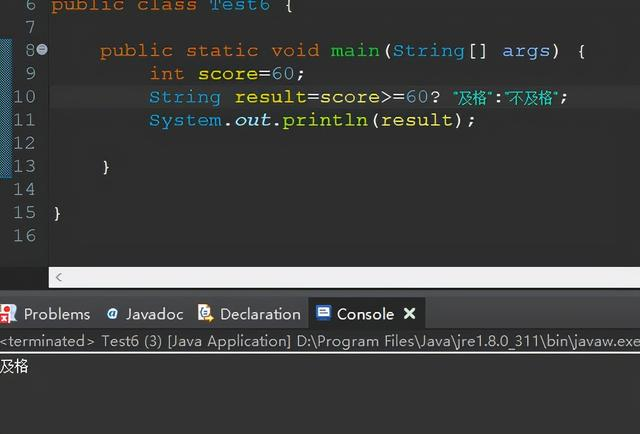instanceof

instanceof运算符的前一个操作数通常是一个引用类型变量，后一个操作数通常是一个类，它用于判断运算符前面的引用变量是否是运算符后面的类型，或其子类型的实例。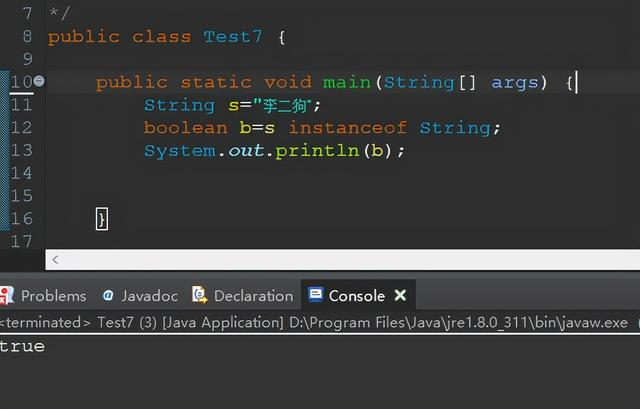运算符 说明 举例 & 与 操作数相同位都为1，那么结果为1 | 或 操作数相同位有一位为1，那么结果为1 ^ 异或 操作数相同位不相同，结果为1 << 左移 各二进制数全部左移N位，高位丢失，低位补0 >> 右移 各二进制位全部右移N位，若值为正，则在高位插入0，若值为负，则在高位插入1 >>> 无符号右移 忽略符号位，空位都以0补齐，正数做>>>运算的时候和>>是一样的，区别在于负数运算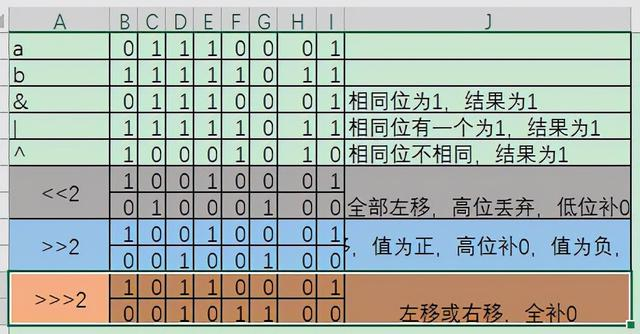顺序 说明 1 括号，如（）和[] 2 一元运算：++、-- 3 算术运算：+ - * / % 4 关系运算：>、<、<=、>=、==、!= 5 逻辑运算：&&、|| 6 条件运算和赋值运算：?:、=、*=、/=、+=、-=